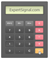Awesome Oscillator

(AO)

Usage: Identifying Momentum

Standard Settings: 5 and 34 Periods SMAsIntroduction to the Awesome Oscillator

The Awesome Oscillator shows the recent market momentum compared to the momentum of a larger number of previous periods. The Awesome Oscillator is an indicator that uses two Simple Moving Average of median prices. The default settings of the Awesome Oscillator involve 2 SMA (5-periods SMA and 34-periods SMA).Calculating The Awesome Oscillator

The Awesome Oscillator is actually a 34-period SMA (simple moving average) subtracted from a 5-period SMA.

■ The Awesome Oscillator = SMA (Median Price of 5 periods) – SMA (Median Price of 34 periods)

Where:

■ The Median Price = ( High Price + Low Price ) / 2Trading with the Awesome Oscillator

The Awesome Oscillator can generate the following trade signals {The original source for the trade signals was Oanda.com}

• The Saucer – This occurs if the bar chart is above the 0-line, and the bar chart has reversed its direction from downward to upward. The saucer occurs if, in any three columns, the second column is lower than the first and colored red and the third column is higher than the second and colored green.
• The Line Crossing - Occurs when the bar chart crosses the 0-line in a positive direction. That is, it passes from the area of negative values to that of positive values.
• Two pikes - Occurs when a pike below the 0-line pointing downwards (the lowest minimum) is followed by another downward-pointing pike that is somewhat higher (closer to the 0-line). This is the only signal to buy that falls below the 0-line.

• The Reversed Saucer – The saucer signal reversed (concave downwards) and below the zero-line.
• The Reversed Line Crossing -The Line Crossing on the decrease - the first column over the 0-line, the second under it.
• Two pikes signals above the 0-line and the pikes pointing upwards (the first one higher than the second).Platform Setup

□ GO TO → INDICATORS → OSCILLATORS → AWESOME OSCILLATOR

□ SETTINGS → 5 AND 34 PERIODS SMAs

Awesome Oscillator (AO)